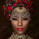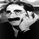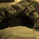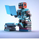64674 views
Here's my version of the MACD _BB . This is a great indicator to capture short term trends.

yellow candles = long
aqua candles = short

This indicator can be much better. I will work on it and publish an improved version (hopefully) soon. In the mean time , go ahead and play around with the code, and please share your findings :)

Cheers

Algo
Open-source script

In true TradingView spirit, the author of this script has published it open-source, so traders can understand and verify it. Cheers to the author! You may use it for free, but reuse of this code in a publication is governed by House Rules. You can favorite it to use it on a chart.

Want to use this script on a chart?
//AK MACD BB
//created by Algokid , February 24,2015

study("AK MACD BB v 1.00")

length = input(10, minval=1, title="BB Periods")
dev = input(1, minval=0.0001, title="Deviations")

//MACD
fastLength = input(12, minval=1)
slowLength=input(26,minval=1)
signalLength=input(9,minval=1)
fastMA = ema(close, fastLength)
slowMA = ema(close, slowLength)
macd = fastMA - slowMA

//BollingerBands

Std = stdev(macd, length)
Upper = (Std * dev + (sma(macd, length)))
Lower = ((sma(macd, length)) - (Std * dev))

Band1 = plot(Upper, color=gray, style=line, linewidth=2,title="Upper Band")
Band2 = plot(Lower, color=gray, style=line, linewidth=2,title="lower Band")
fill(Band1, Band2, color=blue, transp=75,title="Fill")

mc = macd >= Upper ? lime:red

// Indicator

plot(macd, color=mc, style =circles,linewidth = 3)
zeroline = 0
plot(zeroline,color= orange,linewidth= 2,title="Zeroline")

barcolor(macd >Upper ? yellow:na)
//short
barcolor(macd <Lower ? aqua:na)

//needs improvmentsWhat is the difference between the aqua bars with a red boarder and those without - does it signal weak (red boarder) and strong (no boarder)?Great indicator in this market Looking forward to V2 ...I use 5 35 5 setting and scalp aggressivelyHi, modded this indicator a little : added barcolor input ( enable/disable). Thanks !
Source code below:

//AK MACD BB
//created by Algokid , February 24,2015
//modded by Alexandre Pereira, July 6, 2016
color_red_light = #e57373
color_red = #f44336
color_red_dark = #d32f2f

color_blue_light = #64b5f6
color_blue = #2196f3
color_blue_dark = #1976d2

color_green_light = #81c784
color_green = #4caf50
color_green_dark = #388e3c

color_orange_light = #ffb74d
color_orange = #ff9800
color_orange_dark = #f57c00

color_lime_light = #dce775
color_lime = #cddc39
color_lime_dark = #afb42b

study("AK MACD BB v 1.00")

length = input(10, minval=1, title="BB Periods")
dev = input(1, minval=0.0001, title="Deviations")
bar_color = input(false, "Bar colors")
//MACD
fastLength = input(12, minval=1)
slowLength=input(26,minval=1)
signalLength=input(9,minval=1)
fastMA = ema(close, fastLength)
slowMA = ema(close, slowLength)
macd = fastMA - slowMA

//BollingerBands

Std = stdev(macd, length)
Upper = (Std * dev + (sma(macd, length)))
Lower = ((sma(macd, length)) - (Std * dev))

Band1 = plot(Upper, color=color_red_light, style=line, linewidth=2,title="Upper Band")
Band2 = plot(Lower, color=color_green_light, style=line, linewidth=2,title="lower Band")
fill(Band1, Band2, color=color_blue_light, transp=75,title="Fill")

mc = macd >= Upper ? color_green: macd <= Lower ? color_red : color_blue

// Indicator

plot(macd, color=mc, style =circles,linewidth = 3)
zeroline = 0
plot(zeroline,color= orange,linewidth= 2,title="Zeroline")